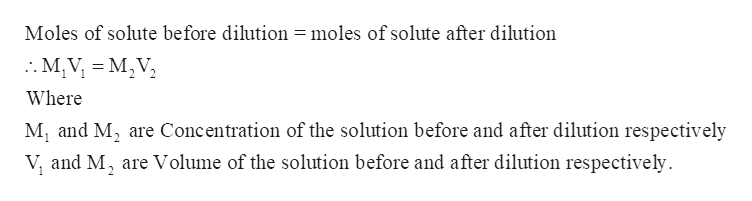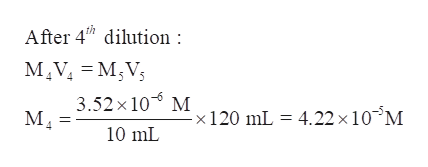# Five standard solutions of HBr are prepared by serial dilution in which, at each stage, 10.00 mL are diluted to 120.00 mL. Given that the concentration of the most dilute solution is 3.52 × 10−6 M, determine the concentration of the original HBr stock solution.

Question
15 views

Five standard solutions of HBr are prepared by serial dilution in which, at each stage, 10.00 mL are diluted to 120.00 mL. Given that the concentration of the most dilute solution is 3.52 × 10−6 M, determine the concentration of the original HBr stock solution.

check_circle

Step 1

Five standard solutions of HBr are prepared by serial dilution in which, at each stage, 10.00 mL are diluted to 120.00 mL. Given that the concentration of the most dilute solution is 3.52 × 10−6 M, the concentration of the original HBr stock solution is to be calculated.

Step 2

The number of moles of solute remains same before the dilution and after the dilution.help_outlineImage TranscriptioncloseMoles of solute before dilution = moles of solute after dilution .M,V M,V Where Mi and M2 are Concentration of the solution before and after dilution respectively V, and M, are Volume of the solution before and after dilution respectively 2 fullscreen
Step 3

Determine the concentration before 5th stage dilution-

Final concentration of 5th solution, M5 = 3.52 × 10−6 M

Volume of the diluted solution, V5 = 120 mL...help_outlineImage TranscriptioncloseAfter 4h dilution: MIVMV 3.52x 10 M120 mL = 4.22 x 10 M М, 10 mL fullscreen

### Want to see the full answer?

See Solution

#### Want to see this answer and more?

Solutions are written by subject experts who are available 24/7. Questions are typically answered within 1 hour.*

See Solution
*Response times may vary by subject and question.
Tagged in

### General Chemistry